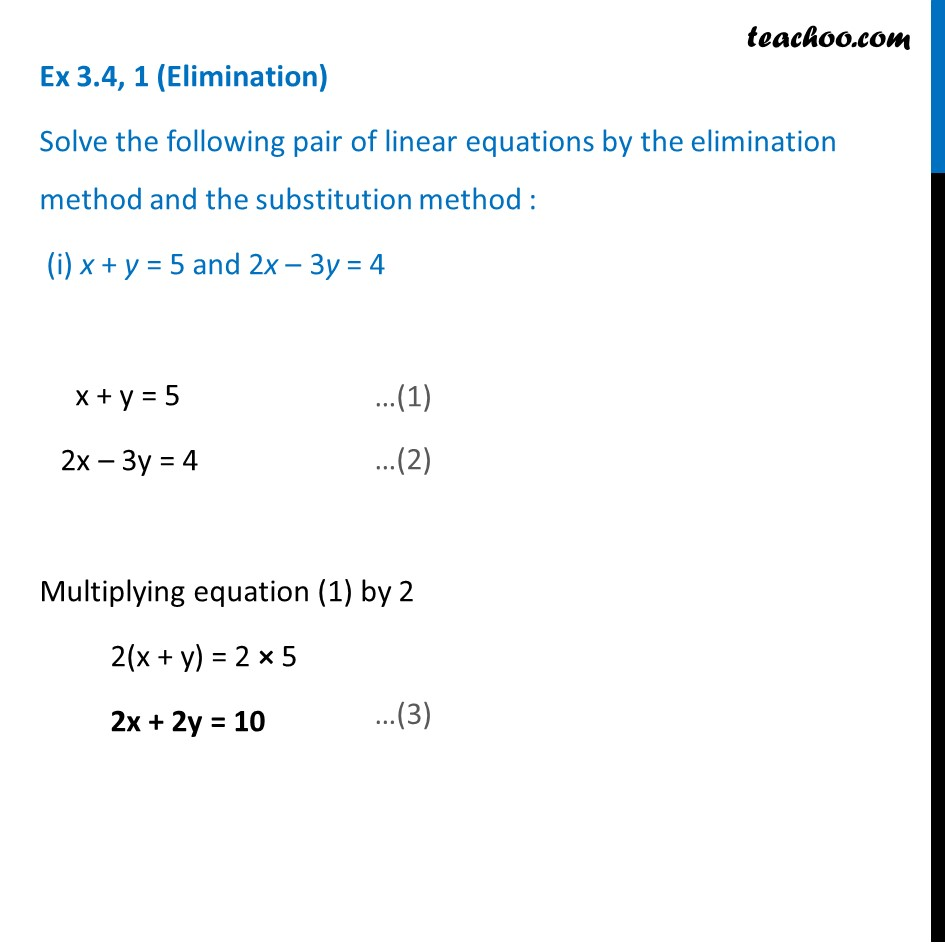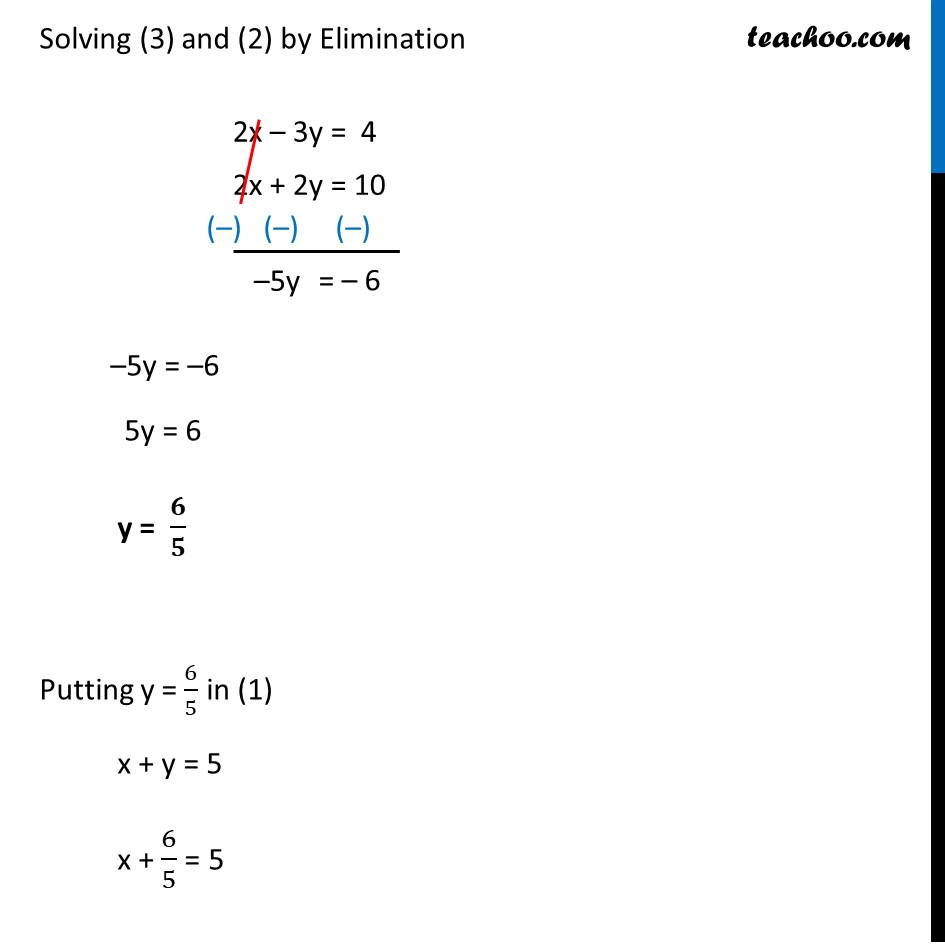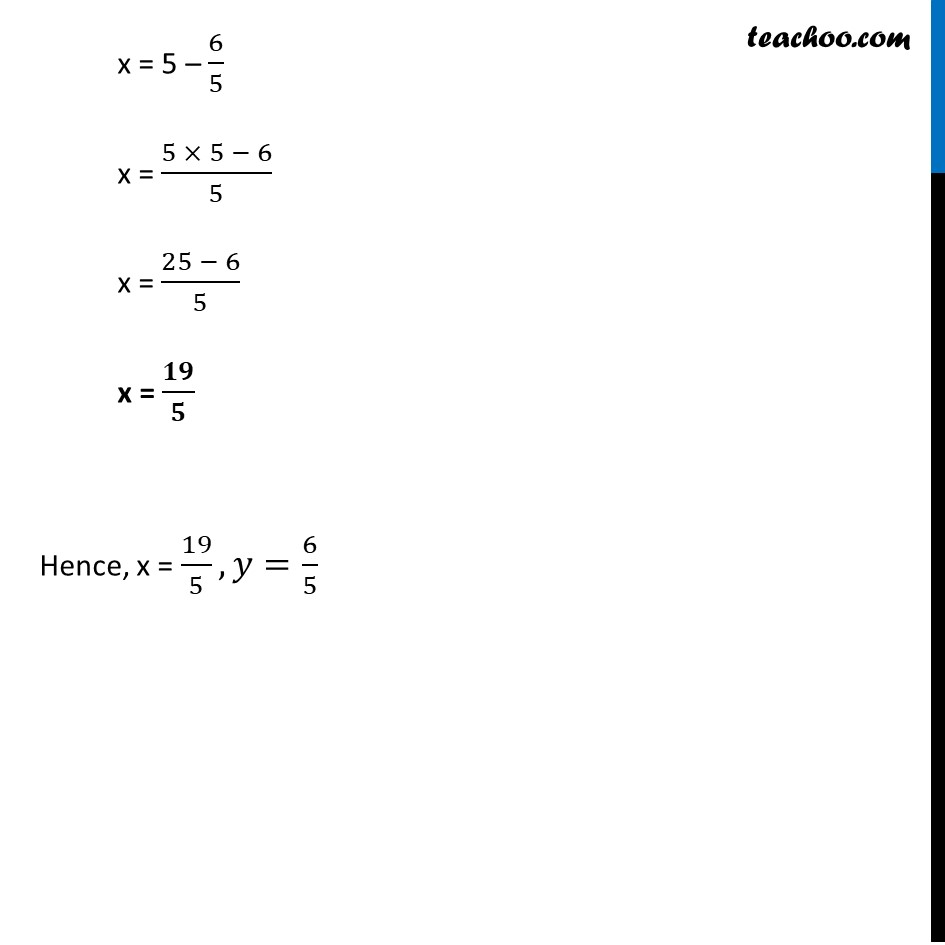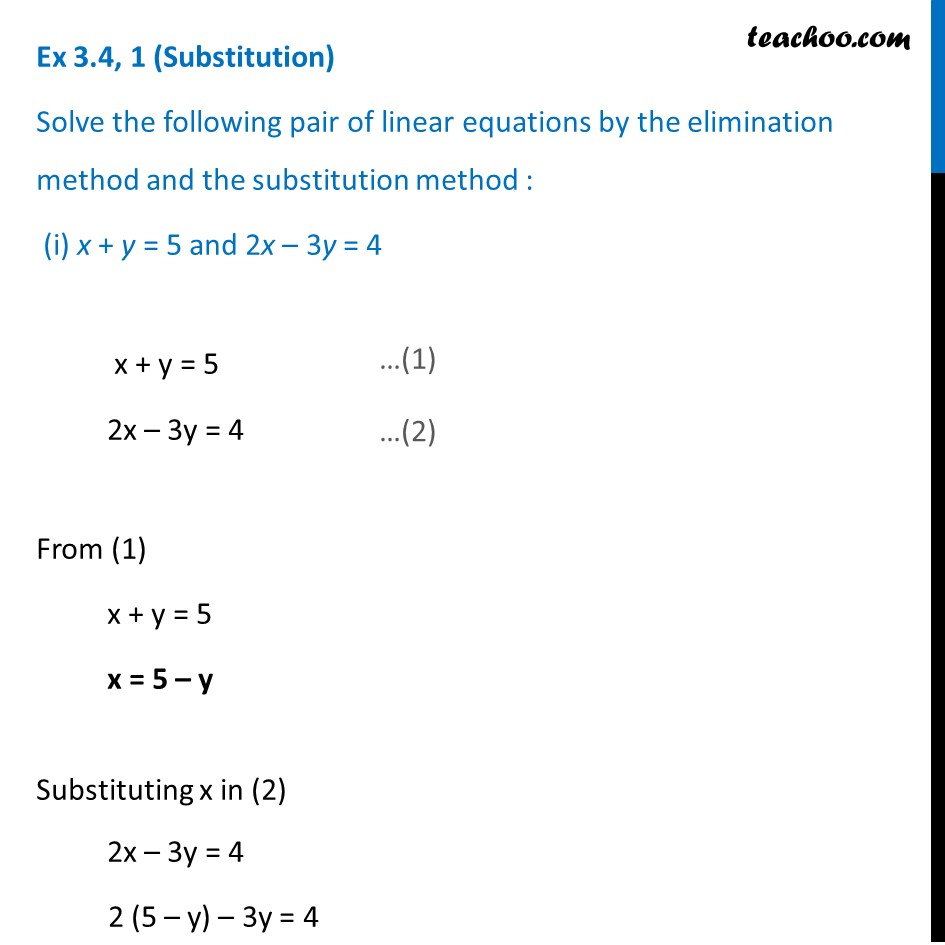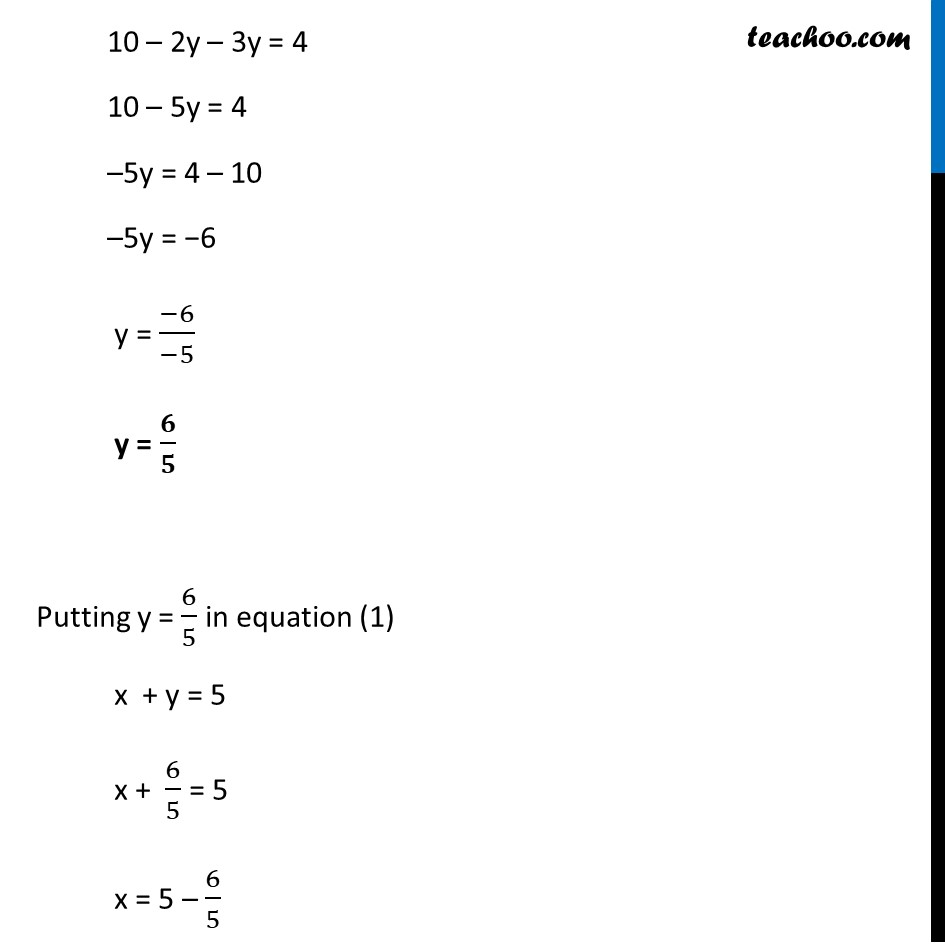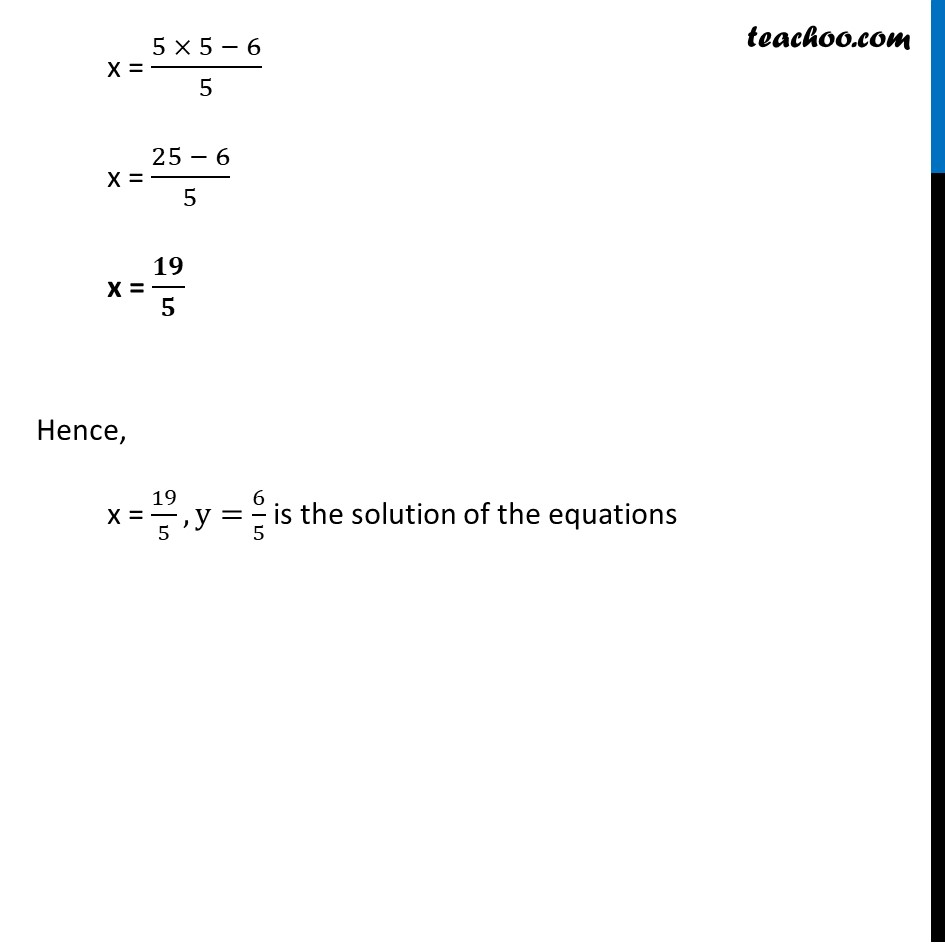1. Chapter 3 Class 10 Pair of Linear Equations in Two Variables (Term 1)
2. Concept wise
3. Elimination

Transcript

Ex 3.4, 1 (Elimination) Solve the following pair of linear equations by the elimination method and the substitution method : (i) x + y = 5 and 2x – 3y = 4 x + y = 5 2x – 3y = 4 Multiplying equation (1) by 2 2(x + y) = 2 × 5 2x + 2y = 10 Solving (3) and (2) by Elimination –5y = –6 5y = 6 y = 𝟔/𝟓 Putting y = 6/5 in (1) x + y = 5 x + 6/5 = 5 x = 5 – 6/5 x = (5 × 5 − 6)/5 x = (25 − 6)/5 x = 𝟏𝟗/𝟓 Hence, x = 19/5,𝑦=6/5 Ex 3.4, 1 (Substitution) Solve the following pair of linear equations by the elimination method and the substitution method : (i) x + y = 5 and 2x – 3y = 4 x + y = 5 2x – 3y = 4 From (1) x + y = 5 x = 5 – y Substituting x in (2) 2x – 3y = 4 2 (5 – y) – 3y = 4 10 – 2y – 3y = 4 10 – 5y = 4 –5y = 4 – 10 –5y = −6 y = (−6)/(−5) y = 𝟔/𝟓 Putting y = 6/5 in equation (1) x + y = 5 x + 6/5 = 5 x = 5 – 6/5 x = (5 × 5 − 6)/5 x = (25 − 6)/5 x = 𝟏𝟗/𝟓 Hence, x = 19/5,y=6/5 is the solution of the equations